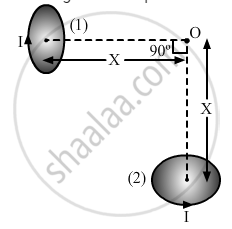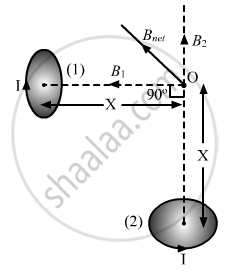Share

# Two Very Small Identical Circular Loops, (1) and (2), Carrying Equal Currents I Are Placed Vertically (With Respect to the Plane of the Paper) with Their Geometrical Axes Perpendicular to Each - Physics

ConceptTorque on a Current Loop in Magnetic Field

#### Question

Two very small identical circular loops, (1) and (2), carrying equal currents I are placed vertically (with respect to the plane of the paper) with their geometrical axes perpendicular to each other as shown in the figure. Find the magnitude and direction of the net magnetic field produced at the point O.#### Solution

The magnetic field at a point due to a circular loop is given by

$B = \frac{\mu_0}{4\pi} . \frac{2\pi I a^2}{\left( a^2 + r^2 \right)^{3/2}}$
where, = current through the loop
a = radius of the loop
r = distance of O from the centre of the loop
Since a and r = x are the same for both the loops, the magnitude of B will be the same and is given by

$B_1 = B_2 = \frac{\mu_0}{4\pi} . \frac{2\pi I a^2}{\left( a^2 + x^2 \right)^{3/2}}$

The direction of magnetic field due to loop (1) will be away from O and that of the magnetic field due to loop (2) will be towards O as shown. The direction of the net magnetic field will be as shown below:The magnitude of the net magnetic field is given by

$B_{net} = \sqrt{{B_1}^2 + {B_2}^2}$

$\Rightarrow B_{net} = \frac{\mu_0}{4\pi} . \frac{2\sqrt{2}\pi I a^2}{\left( a^2 + x^2 \right)^{3/2}}$

Is there an error in this question or solution?

#### Video TutorialsVIEW ALL 

Solution Two Very Small Identical Circular Loops, (1) and (2), Carrying Equal Currents I Are Placed Vertically (With Respect to the Plane of the Paper) with Their Geometrical Axes Perpendicular to Each Concept: Torque on a Current Loop in Magnetic Field.
S